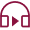# What Do You Mean By Moving Averages In Technical Analysis?Last Updated: 2016-04-11T03:30:00+05:30 ISTMoving averages are most common & simple to use tool for technical analysis. Moving averages do not predict the price direction but rather define the current direction with a lag. That’s why they are called ‘’lagging’’ indicator. Moving averages works well when prices are in trend and it can give false signal when prices are not trending.

For Short term Trend one can use 5, 11 & 21-day moving averages, for the Medium/Intermediate term 21 to 100 days is generally consider as a good measure. Finally any moving average that use 100 days or more, can be consider measuring long term momentum. The shorter the MA the more sensitive is the signal.

## Types of Moving Average:

The most popular types of moving averages are the Simple Moving Average (SMA) and the Exponential Moving Average (EMA). These moving averages can be used to spot the direction of the trend or to identify potential support and resistance levels

## Simple Moving Average (SMA)

A simple moving average is computed by taking the average price of a security over a certain number of periods. While it is also possible to calculate moving averages from the open, the high and the low data points but most moving averages are constructed using the closing price. For example: a 5-day SMA is calculated by adding the closing price for the last 5 days and dividing the total by 5.

Daily closing price 5, 6, 7, 8, 9 & 10

First day of 5-day SMA: (5+6+7+8+9)/5 =7

Second day of 5-day SMA: (6+7+8+9+10)/5 =8

Whether you choose a 21-day average or a 52-week average the calculation is same instead of adding five days you add 21-day or 52 weeks and divide by the same, respectively.

## Exponential Moving Average:

Exponential moving average is used to reduce lag in simple moving average. It reduces the lag by applying more weight to recent prices relative to older prices, hence it will react immediate to recent price change than a SMA For example: a 5 period exponential moving average weighs the most recent price 33.33%

The formula for an exponential moving average is

EMA= (Closing price –EMA (previous day)) * (Multiplier) + EMA (previous day)

A 5-period EMA’s Multiplier is calculated as below:

2/(Time period+1)= 2/(5+1) = 33.33%

## Role Of Moving Average In Determining Market Trends:

Moving average can also be used to determine the trends, If the moving average is rising then the trend is considered up and if the moving average is falling, trend is consider down. It also helps in identifying supports & resistance.

As seen in the following chart of Reliance capital, 100 day medium term moving average which was previously acting as resistance level is now acting as a strong support. Stock also broke 20-day short term moving average several occasions but able to sustain above 100 day moving average, which indicates medium term trend of the stock is very strong. With the combination of two moving averages Investor and investor/trader can make their buy or sell decisions depending upon time horizon to hold.

Role of Moving Averages in Technical Analysis of Market## Moving Average Crossover:

Moving average crossover is popular strategy for defining entry & exit points.

When the shorter moving average crosses above the long term moving average, it shows trend is shifting up and creates buy signal. This is called “Golden Cross”.

When shorter moving average crosses below long term moving average, it shows trend is shifting down and creates sell signal. This is called ‘Death cross.

Moving Average Crossovers – Technical Analysis### Summary:

Moving average helps to identify the trend and keeps you on the right side of the trend, one should not solely rely on the moving average to enter at bottom and exit at top rather it should be used in conjunction with other technical tools that complement them. Moving averages also helps in identifying support & resistance level of security.

### Start Investing in 5 mins*

Get Benefits worth 2100* | Rs. 20 Flat Per Order | 0% Brokerage

### Start Investing in 5 mins*

Get Benefits worth 2100*

Rs. 20 Flat Per Order| 0% Brokerage

SENSEX
59,332.60515.31 (0.88%)
17,534.75
17,534.759.65 (0.06%)
38,287.85
38,287.8550.45 (0.13%)

## Open Free Demat Account

### & get benefits worth 2100*

Resend OTP

By proceeding, you agree to the T&C.

SENSEX
59,332.60515.31 (0.88%)
17,534.75
17,534.759.65 (0.06%)
38,287.85
38,287.8550.45 (0.13%)

### Start Investing Now!

Open Free Demat Account in 5 mins